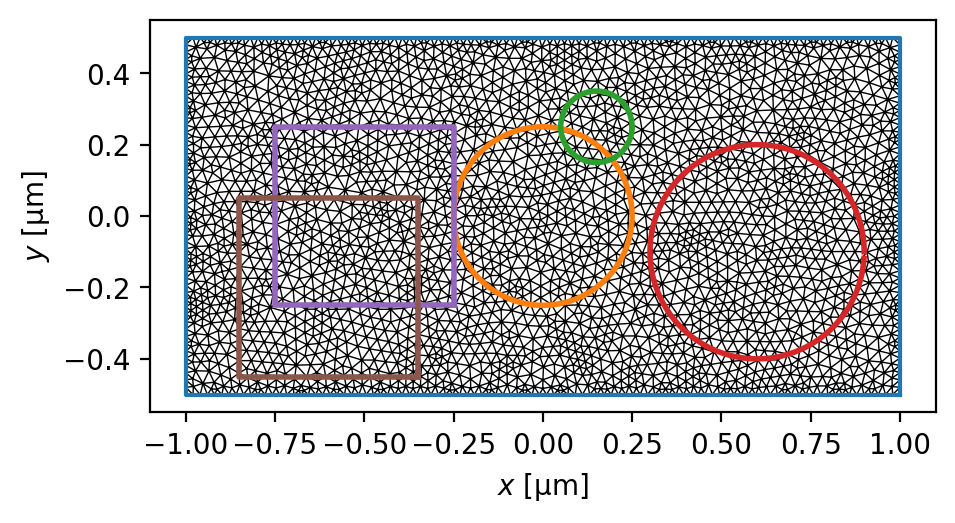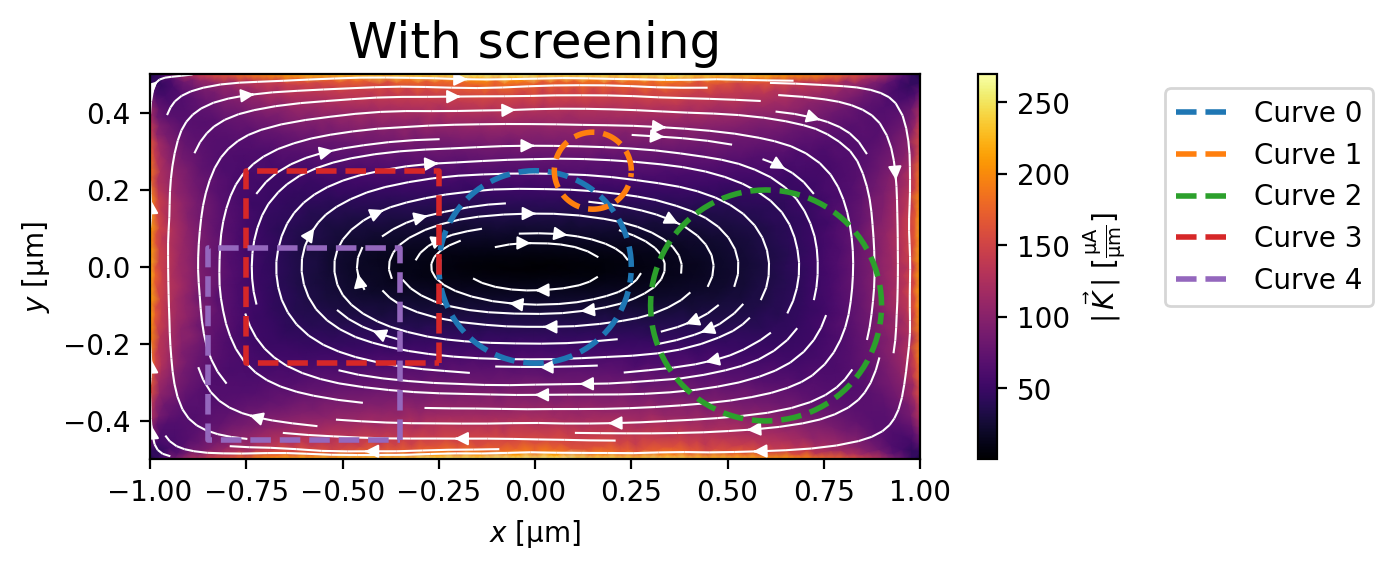# ScreeningBy default pyTDGL assumes that screening is negligible, i.e., that the total vector potential in the film is equal to the applied vector potential: $$\mathbf{A}(\mathbf{r}, t)=\mathbf{A}_\mathrm{applied}(\mathbf{r}, t)$$. Screening can optionally be included by evaluating the vector potential induced by currents flowing in the film. The vector potential in a 2D film induced by a sheet current density $$\mathbf{K}$$ flowing in the film is given by

$\mathbf{A}_\mathrm{induced}(\mathbf{r}, t) = \frac{\mu_0}{4\pi}\int_\mathrm{film}\frac{\mathbf{K}(\mathbf{r}', t)}{|\mathbf{r}-\mathbf{r}'|}\,\mathrm{d}^2\mathbf{r}'.$

Taking the induced vector potential into account, the total vector potential in the film is $$\mathbf{A}(\mathbf{r}, t)=\mathbf{A}_\mathrm{applied}(\mathbf{r}, t)+\mathbf{A}_\mathrm{induced}(\mathbf{r}, t).$$

Because $$\mathbf{A} =\mathbf{A}_\mathrm{applied}+\mathbf{A}_\mathrm{induced}$$ enters into the covariant gradient and Laplacian of $$\psi$$, which in turn determine the current density $$\mathbf{J}=\mathbf{K}/d$$, which determines $$\mathbf{A}_\mathrm{induced}$$, the system must be solved self-consistently at each time step $$t^n$$. The strategy for updating the induced vector potential to converge to a self-consistent value is based on Polyak’s “heavy ball” method:

$\begin{split}\begin{split} \mathbf{A}^{n,s}_{\mathrm{induced},ij} &= \frac{\mu_0}{4\pi}\sum_{\text{sites } \ell}\frac{\mathbf{K}^{n,s}_\ell}{|\mathbf{r}_{ij}-\mathbf{r}_\ell|}a_\ell\\ \mathbf{d}^{n,s}_{ij} &= \mathbf{A}^{n,s}_{\mathrm{induced},ij} - \mathbf{A}^{n,s-1}_{\mathrm{induced},ij}\\ \mathbf{v}^{n,s+1} &= (1-\beta)\mathbf{v}^{n,s} + \alpha\mathbf{d}^{n,s}_{ij}\\ \mathbf{A}^{n,s+1}_{\mathrm{induced},ij} &= \mathbf{A}^{n,s}_{\mathrm{induced},ij} + \mathbf{v}^{n,s+1}_{ij} \end{split}\end{split}$

The integer index $$s$$ counts the number of iterations performed in the self-consistent calculation. The parameters $$\alpha\in(0,\infty)$$ (the screening_step_size) and $$\beta\in(0,1)$$ (the screening_drag) can be set by the user, and the initial conditions for $$\mathbf{A}^{n,0}_{\mathrm{induced},ij} = \mathbf{A}^{n-1}_{\mathrm{induced},ij}$$ and $$\mathbf{v}^{n,0}_{ij} = \mathbf{0}$$. The iterative application of the above equation terminates when the relative change in the induced vector potential between iterations falls below a user-defined tolerance (the screening_tolerance). The screening_step_size $$\alpha$$ and screening_drag $$\beta$$ determine the rate of convergence to the screening_tolerance.

In the equation above, we evaluate the sheet current density $$\mathbf{K}^n_\ell=\mathbf{K}(\mathbf{r}_\ell,t^n)$$ on the mesh sites $$\mathbf{r}_\ell$$ and the vector potential on the mesh edges $$\mathbf{r}_{ij}$$, so the denominator $$|\mathbf{r}_{ij}-\mathbf{r}_\ell|$$ is strictly greater than zero and the equation is well-defined. This method involves the pairwise distances between all edges and all sites in the mesh, so, in contrast to the sparse finite volume calculation, it is a “dense” problem. This means that including screening significantly increases the number of floating point operations required for a TDGL simulation.

A simple way to determine whether screening is non-negligible for a given model is to evaluate the fluxoid for a region inside a film for a small applied field, where the normalized order parameter $$\psi(\mathbf{r})=1$$ everywhere. In this regime, the total fluxoid for a region containing no vortices should exactly vanish, as predicted by the London model. A fluxoid that differs significantly from zero when the model is solved without screening therefore indicates that screening should not be neglected, as demonstrated below.

:

# Automatically install tdgl from GitHub only if running in Google Colab
%pip install --quiet git+https://github.com/loganbvh/py-tdgl.git

:

%config InlineBackend.figure_formats = {"retina", "png"}

import os

import matplotlib.pyplot as plt
import numpy as np

plt.rcParams["figure.figsize"] = (7.5, 2.5)

import tdgl
from tdgl.geometry import box, circle


## Defining the device

Here we define a film with effective magnetic screening length $$\Lambda=\lambda^2/d=(0.075\,\mu\mathrm{m})^2 / (0.05\,\mu\mathrm{m})=0.1125\,\mu\mathrm{m}$$, which is much smaller than the dimensions of the sample. We therefore expect screening to be important for this model.

:

length_units = "um"
xi = 0.1
london_lambda = 0.075
thickness = 0.05

height = 1
width = 2

layer = tdgl.Layer(coherence_length=xi, london_lambda=london_lambda, thickness=thickness)
film = tdgl.Polygon("film", points=tdgl.geometry.box(width, height, points=301))
device = tdgl.Device(
"bar",
layer=layer,
film=film,
length_units=length_units,
)

fluxoid_curves = [
circle(0.25, center=(0, 0)),
circle(0.1, center=(0.15, 0.25)),
circle(0.3, center=(0.6, -0.1)),
box(0.5, center=(-0.5, 0)),
box(0.5, center=(-0.6, -0.2)),
]

:

device.make_mesh(max_edge_length=xi / 2, smooth=100)

Constructing Voronoi polygons: 100%|██████████████████████████████████████████████████████████████████████████| 2862/2862 [00:00<00:00, 22110.78it/s]

:

fig, ax = device.plot(mesh=True, legend=False)
for curve in fluxoid_curves:
ax.plot(*curve.T, lw=2):

device.mesh_stats()

:

Mesh Statistics
num_sites2862
num_elements5426
min_edge_length1.285e-02
max_edge_length4.828e-02
mean_edge_length2.896e-02
min_area9.328e-05
max_area1.446e-03
mean_area6.988e-04
coherence_length1.000e-01
length_unitsum

## Without screening

:

options = tdgl.SolverOptions(
solve_time=5,
field_units="mT",
current_units="uA",
include_screening=False,
)

no_screening_solution = tdgl.solve(device, options, applied_vector_potential=0.1)

Simulating: 100%|███████████████████████████████████████████████████████████████████████████████████████████████████▊| 5/5 [00:00<00:00, 10.00tau/s ]


When screening is neglected, the Meissner supercurrent density is larger than predicted by London theory, meaning that the total vector potential $$\mathbf{A}$$ and supercurrent density $$\mathbf{J}_s$$ are not consistent, leading to a nonzero fluxoid $$\Phi_C$$ for curve $$C$$. In other words, the supercurrent does not “know” that some of the applied magnetic field should have been screened via the Meissner effect, so the supercurrent is responding to a larger magnetic field than would be present in a self-consistent scenario. We can quantify the error in the fluxoid by the ratio

$\begin{split}\begin{split} \Phi_C &= \Phi_C^\mathrm{flux} + \Phi_C^\mathrm{supercurrent}\\ &= \oint_C\mathbf{A}(\mathbf{r})\cdot\mathrm{d}\mathbf{r} +\oint_C\mu_0\Lambda(\mathbf{r})\mathbf{K}_s(\mathbf{r})\cdot\mathrm{d}\mathbf{r}\\ \text{error}&=\left|\frac{\Phi_C^\mathrm{flux} + \Phi_C^\mathrm{supercurrent}}{\Phi_C^\mathrm{flux}}\right|. \end{split}\end{split}$
:

K = no_screening_solution.current_density
K_max = np.sqrt(K[:, 0]**2 + K[:, 1]**2).max()
print(f"Maximum sheet current density: {K_max:.1f~P}")

fig, ax = no_screening_solution.plot_currents()
_ = ax.set_title("No screening", fontsize=18)
for i, curve in enumerate(fluxoid_curves):
fluxoid = no_screening_solution.polygon_fluxoid(curve)
total_fluxoid = sum(fluxoid).magnitude
error = abs(total_fluxoid / fluxoid.flux_part.magnitude)
print(f"Curve {i}: total fluxoid {sum(fluxoid):.3e}, (error {100* error:.1f}%)")
ax.plot(*curve.T, "--", lw=2, label=f"Curve {i}")

_ = ax.legend(bbox_to_anchor=(1.3, 1), loc="upper left")

Maximum sheet current density: 449.9 µA/µm
Curve 0: total fluxoid -1.260e-02 magnetic_flux_quantum, (error 392.6%)
Curve 1: total fluxoid -1.605e-03 magnetic_flux_quantum, (error 1307.5%)
Curve 2: total fluxoid -1.356e-02 magnetic_flux_quantum, (error 8524.0%)
Curve 3: total fluxoid -1.403e-02 magnetic_flux_quantum, (error 651.0%)
Curve 4: total fluxoid -1.019e-02 magnetic_flux_quantum, (error 619.7%)## With screening

:

options = tdgl.SolverOptions(
solve_time=5,
field_units="mT",
current_units="uA",
include_screening=True,
screening_tolerance=1e-6,
dt_max=1e-3,
)

screening_solution = tdgl.solve(device, options, applied_vector_potential=0.1)

Simulating: 100%|███████████████████████████████████████████████████████████████████████████████████████████████████▉| 5/5 [00:54<00:00, 10.89s/tau ]


When screening is included, the supercurrent density is smaller and the fluxoid $$\Phi_C$$ is quantized at 0 $$\Phi_0$$ to within a few percent.

:

K = screening_solution.current_density
K_max = np.sqrt(K[:, 0]**2 + K[:, 1]**2).max()
print(f"Maximum sheet current density: {K_max:.1f~P}")

fig, ax = screening_solution.plot_currents()
_ = ax.set_title("With screening", fontsize=18)

for i, curve in enumerate(fluxoid_curves):
ax.plot(*curve.T, "--", lw=2, label=f"Curve {i}")
fluxoid = screening_solution.polygon_fluxoid(curve)
total_fluxoid = sum(fluxoid).magnitude
error = abs(total_fluxoid / fluxoid.flux_part.magnitude)
print(f"Curve {i}: total fluxoid {sum(fluxoid):.3e}, (error {100 * error:.1f}%)")

_ = ax.legend(bbox_to_anchor=(1.3, 1), loc="upper left")

Maximum sheet current density: 269.5 µA/µm
Curve 0: total fluxoid -7.348e-05 magnetic_flux_quantum, (error 2.0%)
Curve 1: total fluxoid -2.530e-05 magnetic_flux_quantum, (error 3.6%)
Curve 2: total fluxoid -3.784e-05 magnetic_flux_quantum, (error 0.6%)
Curve 3: total fluxoid -5.936e-05 magnetic_flux_quantum, (error 1.1%)
Curve 4: total fluxoid 5.292e-05 magnetic_flux_quantum, (error 0.8%):

tdgl.version_table()

:

SoftwareVersion
tdgl0.8.0; git revision 909069a [2023-10-28]
Numpy1.24.3
SciPy1.10.1
matplotlib3.7.1
cupyNone
numba0.57.1
IPython8.14.0
Python3.10.11 | packaged by conda-forge | (main, May 10 2023, 19:01:19) [Clang 14.0.6 ]
OSposix [darwin]
Number of CPUsPhysical: 10, Logical: 10
BLAS InfoOPENBLAS
Sun Oct 29 16:06:02 2023 PDT
[ ]: